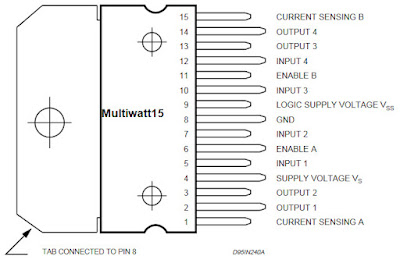# Arduino L298N DC Motor Speed control with PWM

Here the speed and direction of a DC motor is controlled using PWM(Pulse Width Modulation) signal from Arduino. Schematic diagram, DC motor speed control Arduino code, picture and video demonstration are provided. You will learn how to use L298N for DC motor speed control using PWM and how to rotate DC motor in both direction using Arduino.

L298N IC contains two full H-bridge drivers that can output and withstand high voltage and current. It is used to drive equipment which contains inductors such as solenoids, relay and motors. For motor driver application it can drive two DC motors simultaneously or a single stepper motor. In the previous Arduino electronics tutorial How to use L298N motor driver with Arduino, we showed how to drive a simple DC motor using simple code. Here we extend this by including direction and speed control. The following picture shows L298N driver IC.

The following picture shows L298N motor driver pin out taken from L298N datasheet.### Arduino L298N motor driver schematics

The following electrical schematic diagram shows how to connect a DC motor and Arduino with L298N IC.

In the above circuit schematic drawing, the inputs of L298N motor driver IN1(pin 5) and IN2(pin 7) are connected to the pin 10 and pin 9 of Arduino respectively. By sending signal from the Arduino on these L298N pins controls the direction of the motor rotation. The direction is controlled by making pin 10 high and pin 9 low and vice versa. The speed is controlled by sending PWM signal onto the pin 10 or pin 9. The enable ENA pin of the L298N is connected to the digital pin 8 of the Arduino. By sending LOW from the Arduino we can switch off the first dual H-bridge of the L298N and by sending HIGH from Arduino we can turn on the first dual H-bridge of the L298N. The OUT1 and OUT2 of the L298N are connected to two wires of the DC motor. Four 1N4004(or use 1N4001) diodes are connected as shown in the schematic diagram to avoid any unwanted reverse current flowing into the DC motors.

The SenseA and SenseB are for current sensing which are simply connected to ground in this example tutorial. The Vss(or Vcc) pin of the L298N is connected to the +5V logic voltage(can be connected to +5V of the Arduino). The Vs pin of the L298N is connected to the +5V supply which is used to drive the DC motor. This Vs pin should be connected to the higher voltage source in the above L298N motor driver Schematic if higher rated DC motor is used. Here we have used only +5V to drive simple DC motor.The implementation of the above circuit schematic is shown below.

### Arduino code for DC motor speed control with PWM using L298N

Below is Arduino code for DC motor speed control using L298N. Here we have applied PWM signal during the forward and reverse direction.

  //Program for DC motor speed control using PWM with L298N & Arduino
//Source: https://ee-diary.blogspot.com

void forward(int k){
digitalWrite(8,HIGH);
digitalWrite(9,LOW);
analogWrite(10,k);
}

void reverse(int k){
digitalWrite(8,LOW);
digitalWrite(9,HIGH);
analogWrite(10,k);
}

void stop(){
digitalWrite(10, 0);
}

void setup () {
Serial.begin(9600);
pinMode(10,OUTPUT);
pinMode(9,OUTPUT);
pinMode(8,OUTPUT);
digitalWrite(10,0);
}

int sp;
char dir;

void loop() {

Serial.println("\nEnter Direction(f=forward,r=reverse,s=stop):");
while (Serial.available() == 0){}
Serial.println(dir);
while(Serial.available() == 0){}
sp = Serial.parseInt();

switch(dir){

case 'f':
Serial.println("Enter Forward Speed(0 to 255):");
while(Serial.available() == 0){}
sp = Serial.parseInt();
Serial.println(sp);
forward(sp);
break;

case 'r':
Serial.println("Enter Reverse Speed(0 to 255):");
while(Serial.available() == 0){}
sp = Serial.parseInt();
Serial.println(sp);
reverse(sp);
break;

case 's':
Serial.println("motor stopped!");
stop();
break;

default:
while (Serial.available() == 0){}
break;
}

}

In the above arduino pwm motor control example code, user are asked for the direction first. The speed and direction are asked to users on Arduino IDE serial monitor. The user either enters f, r or s for forward direction, reverse direction or to stop the motor.

If the user enters either f or r, then the speed is asked. The speed value can be from 0 to 255. This speed value represents the PWM signal pulse width that is sent to the motor. Larger value means more turn on time and thus more power is delivered to the motor. Depending upon the motor, low value might not cause the motor to rotate. For example in this case, pwm value of 100 or lower will not cause the motor to rotate. In the arduino dc motor speed control code above, we have used forward(), reverse() and stop() function that gets called for forward rotation or backward rotation with specified pwm value or to stop the motor. We have used Serial.ParseInt() function to read integer from IDE serial monitor for PWM value. This read value is passed into the forward() and reverse() function for speed control of a DC motor using Arduino. Also in the arduino code for dc motor control above, we have used Serial.available() == 0 statement to wait for user input.The following video demonstrates the speed and direction control of DC motor using Arduino and L298N motor driver.